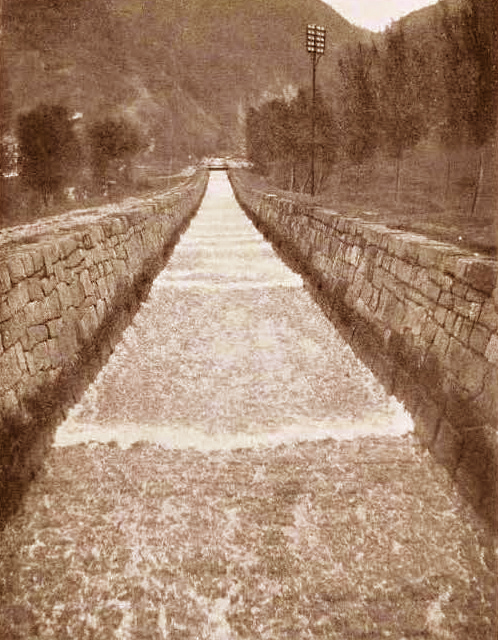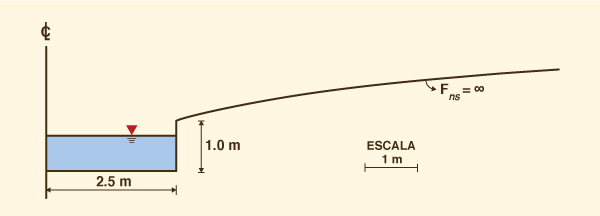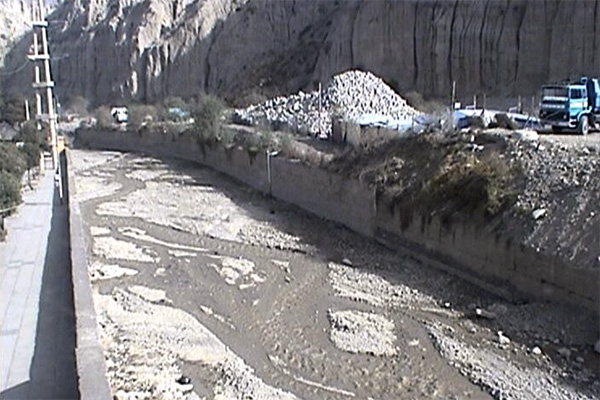Design of a stable channelwith a steep slope usingthe exponent of the rating curve Victor M. Ponce24 February 2020

 Abstract. The design of a lined channel, with a steep slope, to be hydraulically stable is governed by the well-known Vedernikov criterion. However, it can be shown that this depends on the shape of the cross section, whether trapezoidal, rectangular, or triangular. For a given section, there is a unique relationship between the exponent β of the rating curve Q - A (discharge vs flow area), and the value of V /F, in which V = Vedernikov number, and F = Froude number. In this work we use the onlinechannel15b calculator to calculate the value of β and the corresponding Vedernikov number for a rectangular, trapezoidal, or triangular cross section. Three series of tests are carried out in a hypothetical channel, keeping constant discharge Q, Manning's n, and bottom slope S, and varying the value of the side slope z: (a) 0.25; (b) 0.5, and (c) 1. It is concluded that when the bottom width b is reduced, the Vedernikov number V is reduced more quickly to values less than 1 for the lower values of z in the range 0.25 ≤ z ≤ 1.

1.  INTRODUCTION

The design of a lined channel, with a steep slope, to be hydraulically stable is governed by the well-known Vedernikov criterion (Ponce, 2014). However, it can be shown that this depends on the shape of the cross section, whether trapezoidal, rectangular, or triangular. For a given section, there is a unique relationship between the exponent β of the rating curve Q - A (discharge vs flow area), and the value of V/F, in which V = Vedernikov number, and F = number of Froude number.

In this work we calculate the value of β corresponding to a section of trapezoidal, rectangular, or triangular shape, using an online calculator. Unstable flow theory indicates that stability is achieved for values of β somewhat larger but close to 1. At the lower limit, for β = 1, the section is inherently stable, that is, stable for any value of the Froude number (Ponce and Porras, 1995). Here we calculate the value of β for a series of sections, keeping the flow Q and bottom slope S constant, and varying the lateral slope (slope) z (z H : 1 V) and the depth of flow y.

The calculator determines the numbers of Froude F and Vedernikov V, and the associated value of β. The optimal design section is the one that corresponds to the lowest value of β compatible with the planned cost of the project. The latter is a function of the depth of excavation required to ensure that the flow remains stable, that is, so that V < 1.

2.  THEORETICAL BACKGROUND

The theory of hydrodynamic stability of flow in open channels is due to Vedernikov, who in 1945 introduced the concept of the number bearing his name (Vedernikov, 1945; Powell, 1948). According to this theory, the Vedernikov number is the relationship between the relative celerity of the kinematic wave and the relative celerity of the dynamic wave (Ponce, 1991). For V ≤ 1, the flow is stable; for V > 1 the flow is unstable. The latter is associated with the so-called roll waves, or pulsating waves (Fig. 1).Cornish
 Fig. 1  Roll waves in a channel of the Swiss Alps (Cornish, 1907).

In certain cases, the pulsating waves can be of such magnitude to jeopardize human safety and property, as evidenced by recent experience in some channeled rivers of La Paz, Bolivia (Fig. 2) (Ponce and Choque, 2019). Therefore, it is imperative to design river channels to avoid (decrease or minimize) the incidence of roll waves. This objective can be achieved by designing the cross section in order to reduce the value of β so that the number of Vedernikov for the adopted section is less than 1.Courtesy of Jorge Molina
 Fig. 2  Pulsating wave on the Huay�ajahuira river, La Paz, Bolivia (2016).

3.  RELATIONSHIP BETWEEN β and V /F

Ponce (2014) has determined the relationship between the exponent β of the expenditure curve (flow Q vs flow area A) and the ratio V /F:

 V β   =   1  +   _____                       F
(1)

For V = 1, the neutrally stable Froude number Fne is:

 1 Fne   =     _____                β - 1
(2)

Table 1 shows the asymptotic values of β and Fne corresponding to three types of cross section and two types of friction in the turbulent flow regime. Figure 3 shows the shape of the inherently stable section (Liggett, 1975; Ponce and Porras, 1995).

 Table 1. Values of β and Fne corresponding. Cross section shape Friction type β Fne Hydraulically wide Manning 5/3 3/2 Chezy 3/2 2 Triangular Manning 4/3 3 Chezy 5/4 4 Inherently stable Manning or Chezy 1 ∞Fig. 3  The inherently stable section (Ponce and Porras, 1995).

4.  ONLINE CALCULATOR

The canalenlinea15b calculator, written in the PHP language, calculates the value of β corresponding to a rectangular, trapezoidal, or triangular section. The input data is:

1. Bottom width b

2. Flow depth y

3. Side slope z1

4. Side slope z2

5. Manning coefficient n

6. Bottom slope S.

The output results are:
1. Flow (discharge) Q

2. Flow rate v

3. Hydraulic depth D

4. Top width T

5. Froude Number F   [F = v /(gD1/2)]

6. Exponent of the rating curve β

7. Neutrally stable Froude number Fne

8. Vedernikov number V.

Given a preselected flow discharge Q, and setting the values of z1, z2, n and S, the objective is to vary the bottom width b (of the trapezoidal channel) in a reasonable range, to calculate y, v, F, β, Fne and V. The results are tabulated to determine the most appropriate bottom width for the design, taking into account the Vedernikov criterion.

5.  THE UNSTABLE CHANNEL

The unstable channel is of rectangular section, of width b = 6 m. The design discharge is Q = 100 m3/s; Manning coefficient n = 0.025 (masonry lining); and the bottom slope S = 0.06. This data simulate approximately the conditions existing in the channelized Achumani and Huay�ajahuira rivers of La Paz, Bolivia (Figs. 4 and 5). In these channels, the occurrence of pulsating wave events has been documented with some regularity (Ponce and Choque, 2019).Fig. 4  The channelized Achumani river, La Paz, Bolivia.Fig. 5  The channelized Huay�ajahuira river , La Paz, Bolivia.

The result of the calculation using canalenlinea15b is shown in Fig. 6. Note that for this unstable rectangular channel, the Vedernikov number is V = 1.48.Fig. 6  Calculation of the unstable channel.

6.  TEST PROGRAM

The objective of the test program is to determine the hydraulic conditions in a series of alternative trapezoidal sections for which the Vedernikov number falls below V = 1. This is obtained by reducing the bottom width b and specifying a trapezoidal section (z > 0).

The program considers three series of trapezoidal sections:

• z = 0.25;

• z = 0.5;

• z = 1.0.

The calculation results are shown in Tables 2 to 4. It is concluded that when the bottom width b is reduced in the range 5 ≥ b ≥ 1, the Vedernikov number V is reduced more quickly to values less than 1 for lower values of the lateral slope z in the range 0.25 ≤ z ≤ 1. Note that the lowest value V = 0.55 is obtained for the case z = 0.25 and b = 1 (Table 2).

 Table 2. Results for Series A:  z = 0.25. Variable Bottom width b (m) 5 4 3 2 1 y 1.754 2.078 2.581 3.408 4.769. v 10.48 10.65 10.63 10.29 9.569 D 1.623 1.863 2.192 2.624 3.989 T 5.877 5.039 4.290 3.704. 3.384 F 2.62 2.49 2.29 2.02 1.73 β 1.56 1.53 1.48 1.40 1.32 Fne 1.76 1.87 2.07 2.45 3.12 V 1.48 1.32 1.10 0.82 0.55

 Table 3. Results for Series B:  z = 0.5. Variable Bottom width b (m) 5 4 3 2 1 y 1.643 1.894 2.249 2.763 3.503 v 10.45 10.67 10.78 10.70 10.38 D 1.439 1.589 1.767 1.961 2.140 T 6.643 5.894 5.249 4.763 4.503 F 2.78 2.70 2.59 2.44 2.26 β 1.56 1.53 1.49 1.43 1.35 Fne 1.76 1.86 2.02 2.30 2.77 V 1.57 1.44 1.27 1.05 0.81

 Table 4. Results for Series C:  z = 1. Variable Bottom width b (m) 5 4 3 2 1 y 1.509 1.689 1.922 2.224 2.613 v 10.18 10.40 10.57 10.64 10.59 D 1.225 1.302 1.382 1.456 1.516 T 8.018 7.378 6.844 6.448 6.226 F 2.93 2.91 2.87 2.81 2.74 β 1.55 1.52 1.49 1.44 1.38 Fne 1.79 1.89 2.03 2.25 2.61 V 1.63 1.54 1.41 1.24 1.05

7.  ANALYSIS

It should be noted that the Froude number F is a function of hydraulic depth D (and not of flow depth y) (See Section 4). The examination of Tables 2 to 4 leads to the following conclusions:

1. As z decreases, D increases and, therefore, F decreases.

2. The values of V / F, and therefore those of β (Eq. 1), decrease with the value of z.

3. The neutrally stable Froude Fne and, consequently, hydrodynamic stability (V < 1), increase with decreasing β (in the range β ≥ 1).

4. For the lower values of z, the Vedernikov number falls below 1.

8.  CONCLUSIONS

The results of Tables 2 to 4 show that as the bottom width b decreases in the range 5 ≥ b ≥ 1, the values of β and V also decrease progressively. The reduction in the Vedernikov number depends on the lateral slope z, the latter being faster with the decrease of the bottom width b, when the slope z is smaller. That is, when the value of z is lower (in the range 0.25 ≤ z ≤ 1), the faster the Vedernikov number falls below 1, resulting in the flow becoming stable faster.

The canalenlinea15b calculator is a very useful tool for the analysis and design of the cross-section of channelized rivers, with the aim of promoting stable flow and, therefore, avoid roll waves.

REFERENCES

Cornish, V. 1907. Progressive waves in rivers. Journal of the Royal Geographical Society, Vol. 29, No. 1, January, 23-31.

Liggett, J. A. 1975. Stability. Chapter 6 in Unsteady Flow in Open Channels, K. Mahmood and V. Yevjevich, eds., Water Resources Publications, Ft. Collins, Colorado.

Ponce, V. M. 1991. New perspective on the Vedernikov number. Water Resources Research, Vol. 27, No. 7, 1777-1779, July.

Ponce, V. M., y P. J. Porras. 1995. Effect of cross-sectional shape on free-surface instability. Journal of Hydraulic Engineering, ASCE, Vol. 121, No. 4, April, 376-380.

Ponce, V. M. 2014. Chow, Froude, and Vedernikov. Proceedings, American Society of Civil Engineers (ASCE) World Environment and Water Resources Congress, June 1-5, 2014, Portland, Oregon.

Ponce, V. M. y B. Choque Guzmán, 2019. El control de ondas pulsantes en ríos canalizados. http://ponce.sdsu.edu/el_control_de_ondas_pulsantes.html   [Cited on February 16, 2020].

Powell, R. W. 1948. Vedernikov's criterion for ultra-rapid flow. Transactions, American Geophysical Union, Vol. 29, No. 6, 882-886.

Vedernikov, V. V. 1945. Conditions at the front of a translation wave disturbing a steady motion of a real fluid, Dokl. Akad. Nauk. SSSR, 48(4), 239-242.

 200224 17:45